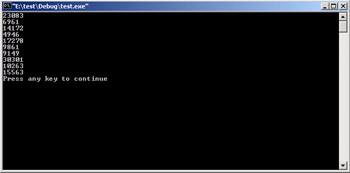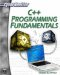# Random Numbers

There are many cases where you will wish to generate a random number. If you wished to generate a random number to use as an encryption key, or if you needed random numbers for a game, you would require some function that generates a random number for you.

There are actually two functions you will need to know about. The first is rand(). This function will only return a pseudo random number. The way to fix this is to first call the srand() function. That function seeds the rand() function with a truly random number to start it off. Usually you use the time function to get the number of seconds on your system time, to randomly seed the rand() function. The following example demonstrates this.

### Example 4.11

Step 1: Place the following code in your favorite text editor.

`#include <iostream> #include <ctime> using namespace std; void main( void ) {  int i,j;  srand( (unsigned)time( NULL ) );  /* Display 10 numbers. */  for( i = 0;i < 10;i++ )  {    j= rand();    cout << j << endl;      } }`

Step 2: Compile and execute the code. You should see something like what is depicted in Figure 4.11Figure 4.11: Using the random functions.

The most important thing to remember is that you must use the srand() function to seed the random function or you will not get truly random numbers. Later in this text when we develop a simple game, you will see the random function used.C++ Programming Fundamentals (Cyberrookies)
ISBN: 1584502371
EAN: 2147483647
Year: 2005
Pages: 197
Authors: Chuck Easttom

flylib.com © 2008-2017.
If you may any questions please contact us: flylib@qtcs.net